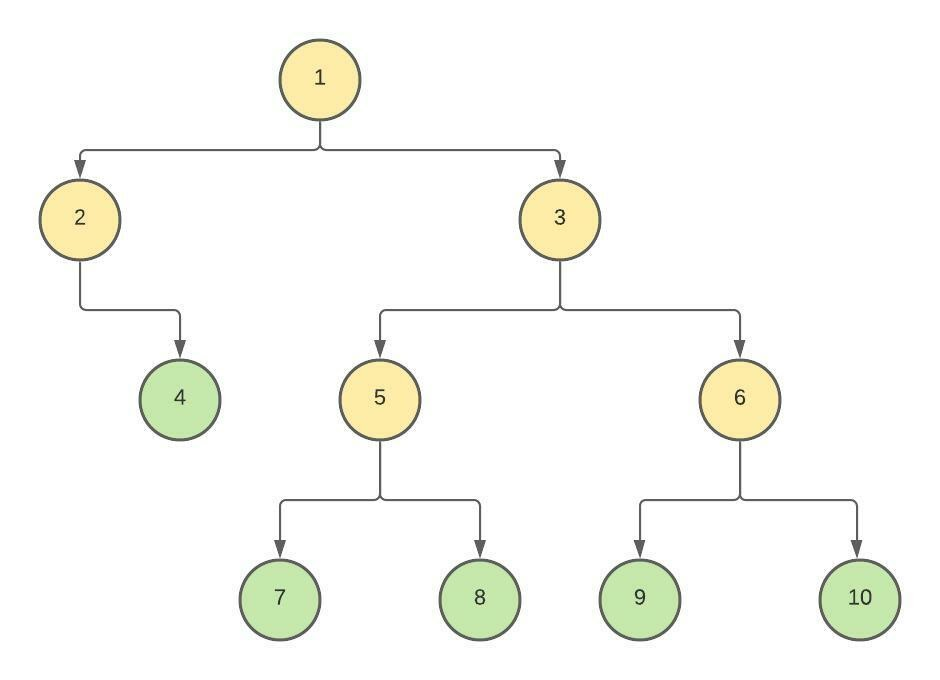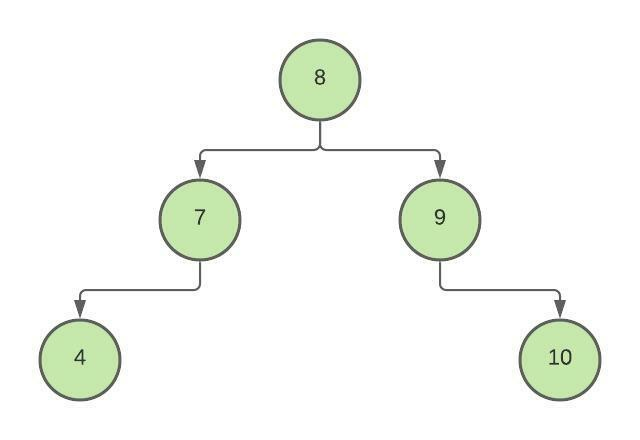# Create a Balanced Binary Tree using leaf Nodes of a Binary Tree without using Extra Space

## Introduction-

This article will discuss how to create a balanced binary tree using leaf nodes of a binary tree without using extra space. Before moving further, let's see what a balanced binary tree is.

"A balanced binary tree, also known as a height-balanced binary tree, is a special type of binary tree in which the height of left subtree and right subtree of any node differs maximum by 1."
In this problem, we will be given a binary tree as input, and we have to create a balanced binary tree using leaf nodes of a binary tree that is given to us, and we have to do this without using any extra space.

Let's see the below example to understand this.

Suppose the following binary tree is given to us:In the above example, we can see that the nodes shown in green color are the leaf nodes. Using these leaf nodes, the following balanced binary tree can be formed:## Solution Approach

In this article, we are going to discuss how to create a balanced binary tree using leaf nodes of a binary tree without using any extra space. The idea is to first create a doubly linked list using the leaf nodes of the binary tree and then create a balanced binary tree using that doubly linked list.

We will have to create two recursive functions. The first one is to create a doubly linked list from leaf nodes of the binary tree. In this function, traverse the binary tree, and when a leaf node is reached, add that node into the doubly linked list and remove it from the binary tree. Then a second function to create a balanced binary tree using the doubly linked list. In this function, make the middle node of the doubly linked list as the root of the balanced binary tree and then recursively build its left and right subtree using the first half and last half nodes of the doubly linked list.

## Algorithm -

Step 1. Create a class "Node" for creating the tree and doubly linked list.

Step 2. Inside the "main" function, construct a binary tree using a node constructor and then call a function to create a doubly linked list from the leaf nodes of the binary tree.

Step 3. Create a recursive function "createLeafLinkedList()" to create a doubly linked list from the leaf nodes of a binary tree. It will take two inputs - the root node of the binary tree and pointer to the head node pointer of the doubly linked list. Inside the function, write the base case that if the node is null, then return null. Then check if the node is a leaf node, then add that node to the doubly linked list and return null to remove that node from the binary tree. After that, call the function recursively for the left and right subtree of the node. Note that we don't have to use any extra space, so we are not creating any nodes but using the same nodes and joining them to create the doubly linked list.

Step 4.  Now, inside the "main" function, we have got the doubly linked list created from the leaf nodes, now call a function to count the number of nodes in the doubly linked list, then call a recursive function to construct the balanced binary tree using the doubly linked list.

Step 5. Next, create a "count()" function to count the number of nodes in the doubly linked list. It takes the head of the doubly linked list as input and returns the count of nodes.

Step 6.  Next, create a function "DLLToBBT()" to create a balanced binary tree using a doubly linked list. It takes two inputs - pointer to the head pointer of the doubly linked list and the number of nodes in the linked list and returns the root of the balanced binary tree. Write the base case that if a number of nodes are zero, then return null. Now call the function recursively to create the left subtree by using the first half nodes and then use the middle node to create the root node and then move the head node to the right child and call the function recursively to create the right subtree using the last half nodes.

Step 6. Finally, create the "inorder()" function to print the inorder traversal of the resultant balanced binary tree.

## Algorithm Complexity:

Time Complexity: O(N)

In the above code, to create a balanced binary tree using leaf nodes of a binary tree, we have created four functions. Each of the four functions traverses to each node and takes O(1) time for each node. So, the overall time complexity will be O(N), where 'N' is the number of nodes in the binary tree.

Space Complexity: O(1)

In the above code, to create a balanced binary tree using leaf nodes of a binary tree, we have used constant space, so the space complexity will be O(1).

1. What is a balanced binary tree?

A balanced binary tree, also known as a height-balanced binary tree, is a special type of binary tree in which the height of the left subtree and right subtree of any node differs maximum by 1.

2. What is a doubly-linked list?

A doubly linked list is a linear data structure formed by linearly connected nodes. Each node has a value stored in it and the address of the previous and next nodes.

## Key takeaways-

In this article, we discussed how to create a balanced binary tree using leaf nodes of a binary tree without using any extra space., its C++ implementation, and its time and space complexity. If you want to solve more problems on tree data structure for practice, you can visit codestudio.

If you think that this blog helped you share it with your friends!. Refer to the DSA C++ course for more information.

Until then, All the best for your future endeavors, and Keep Coding.